Hunt-Stein theorem

A theorem stating conditions under which there exists a maximin invariant test in a problem of statistical hypothesis testing.

Suppose that based on the realization of a random variabletaking values in a sampling space,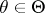, it is necessary to test a hypothesis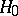: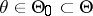against an alternative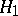: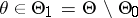, and it is assumed that the familyis dominated by a certain-finite measure(cf. Domination). Next, suppose that ona transformation group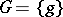acts that leaves invariant the problem of testing the hypothesisagainst, and letbe the Borel-field of subsets of. The Hunt–Stein theorem asserts that if the following conditions hold:

1) the mapping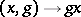is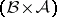-measurable andfor every set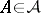and any element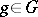;

2) onthere exists an asymptotically right-invariant sequence of measuresin the sense that for anyand,then for any statistical test intended for testingagainstand with critical function, there is an (almost-) invariant test with critical functionsuch that for all,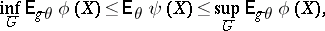whereis the group induced by.

The Hunt–Stein theorem implies that if there exists a statistical test of levelwith critical function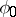that maximizes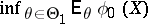, then there also exists an (almost-) invariant test with the same property.

Condition 2) holds necessarily whenis a locally compact group on which a right-invariant Haar measure is given. The Hunt–Stein theorem shows that ifsatisfies the conditions of the theorem, then in any problem of statistical hypothesis testing that is invariant relative toand on which there exists a uniformly most-powerful test, this test is a maximin test.

Conversely, suppose that in some problem of statistical hypotheses testing that is invariant under a groupit is established that a uniformly most-powerful test is not a maximin test. This means that the conditions of the Hunt–Stein theorem are violated. In this connection there arises the question: Can a given test be maximin in another problem of hypothesis testing that is invariant under the same group? The answer to this question depends not only on the group, but also on the family of distributions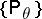itself.

The theorem was obtained by G. Hunt and C. Stein in 1946, see .